# cavityCircular

Create circular cavity-backed antenna

## Description

Use the `circularCavity` object to create a circular cavity-backed antenna. By default, the exciter used is a dipole. The dimensions are chosen for an operating frequency of 1 GHz..

## Creation

### Syntax

``circularcavity = cavityCircular``
``circularcavity = cavityCircular(Name=Value)``

### Description

example

````circularcavity = cavityCircular` creates a circular cavity-backed antenna. ```

example

````circularcavity = cavityCircular(Name=Value)` sets properties using one or more name-value pairs. For example, `circularcavity = cavityCircular(Radius=0.2)` creates a circular cavity of radius 0.2 m. Enclose each property name in quotes.```

## Properties

expand all

Exciter antenna or array type, specified as a single-element antenna object, an array object, or an empty array. Except for reflector and cavity antenna elements, you can use any Antenna Toolbox™ antenna or array element as an exciter. To create the cavity backing structure without the exciter, specify this property as an empty array.

Example: `horn`

Example: `linearArray(Element=patchMicrostrip)`

Example: `[]`

Radius of cavity, specified as a scalar in meters.

Example: `0.2`

Data Types: `double`

Cavity height along z-axis, specified as a scalar in meters.

Example: `0.001`

Data Types: `double`

Distance between the exciter and the base of the cavity, specified a scalar in meters.

Example: `7.5e-2`

Data Types: `double`

Type of dielectric material used as a substrate, specified as a dielectric object. For more information see, `dielectric`. For more information on dielectric substrate meshing, see Meshing.

Note

The substrate dimensions must be equal to the ground plane dimensions.

Example: `dielectric("FR4")`

Create probe feed from backing structure to exciter, specified as `0` or `1` or a positive scalar. By default, probe feed is not enabled.

Example: `1`

Data Types: `logical`

Type of the metal used as a conductor, specified as a metal material object. You can choose any metal from the `MetalCatalog` or specify a metal of your choice. For more information, see `metal`. For more information on metal conductor meshing, see Meshing.

Example: `metal("Copper")`

Lumped elements added to the antenna feed, specified as a lumped element object. For more information, see `lumpedElement`.

Example: `lumpedElement(Impedance=75)`

Tilt angle of the antenna, specified as a scalar or vector with each element unit in degrees. For more information, see Rotate Antennas and Arrays.

Example: `Tilt=90`

Example: `Tilt=[90 90]`,`TiltAxis=[0 1 0;0 1 1]` tilts the antenna at 90 degrees about the two axes defined by the vectors.

Note

The `wireStack` antenna object only accepts the dot method to change its properties.

Data Types: `double`

Tilt axis of the antenna, specified as:

• Three-element vector of Cartesian coordinates in meters. In this case, each coordinate in the vector starts at the origin and lies along the specified points on the X-, Y-, and Z-axes.

• Two points in space, each specified as three-element vectors of Cartesian coordinates. In this case, the antenna rotates around the line joining the two points in space.

• A string input describing simple rotations around one of the principal axes, 'X', 'Y', or 'Z'.

Example: `TiltAxis=[0 1 0]`

Example: `TiltAxis=[0 0 0;0 1 0]`

Example: `TiltAxis = 'Z'`

Data Types: `double`

## Object Functions

 `show` Display antenna, array structures or shapes `axialRatio` Axial ratio of antenna `beamwidth` Beamwidth of antenna `charge` Charge distribution on antenna or array surface `current` Current distribution on antenna or array surface `design` Design prototype antenna or arrays for resonance around specified frequency `efficiency` Radiation efficiency of antenna `EHfields` Electric and magnetic fields of antennas; Embedded electric and magnetic fields of antenna element in arrays `impedance` Input impedance of antenna; scan impedance of array `mesh` Mesh properties of metal, dielectric antenna, or array structure `meshconfig` Change mesh mode of antenna structure `optimize` Optimize antenna or array using SADEA optimizer `pattern` Radiation pattern and phase of antenna or array; Embedded pattern of antenna element in array `patternAzimuth` Azimuth pattern of antenna or array `patternElevation` Elevation pattern of antenna or array `rcs` Calculate and plot radar cross section (RCS) of platform, antenna, or array `returnLoss` Return loss of antenna; scan return loss of array `sparameters` Calculate S-parameter for antenna and antenna array objects `vswr` Voltage standing wave ratio of antenna

## Examples

collapse all

Create and view a default circular cavity-backed antenna.

`a = cavityCircular`
```a = cavityCircular with properties: Exciter: [1x1 dipole] Substrate: [1x1 dielectric] Radius: 0.1000 Height: 0.0750 Spacing: 0.0750 EnableProbeFeed: 0 Conductor: [1x1 metal] Tilt: 0 TiltAxis: [1 0 0] Load: [1x1 lumpedElement] ```
`show(a)`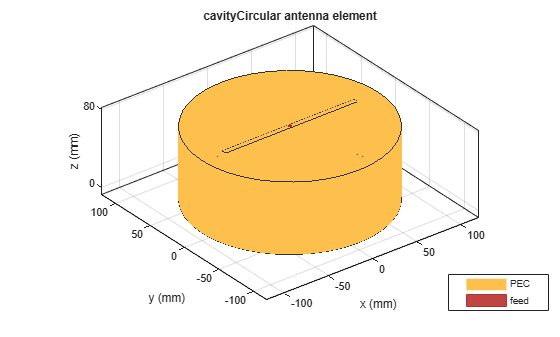Create and view an equiangular spiral backed by a circular cavity. The cavity dimensions are:

Height = 0.01 m

Spacing = 0.01 m

``` ant = cavityCircular(Exciter=spiralEquiangular,Radius=0.02, ... Height=0.01,Spacing=0.01); show(ant) ```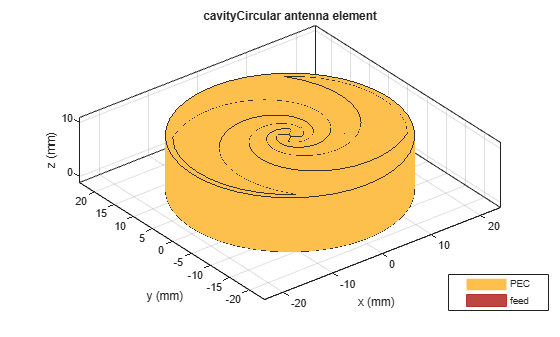Create a linear array of H-shaped patch microstrip antenna.

`arr = linearArray(Element=patchMicrostripHnotch,ElementSpacing=0.04);`

Create a circular cavity-backed antenna with linear array exciter.

`ant = cavityCircular(Exciter=arr)`
```ant = cavityCircular with properties: Exciter: [1x1 linearArray] Substrate: [1x1 dielectric] Radius: 0.1000 Height: 0.0750 Spacing: 0.0750 EnableProbeFeed: 0 Conductor: [1x1 metal] Tilt: 0 TiltAxis: [1 0 0] Load: [1x1 lumpedElement] ```
`show(ant)`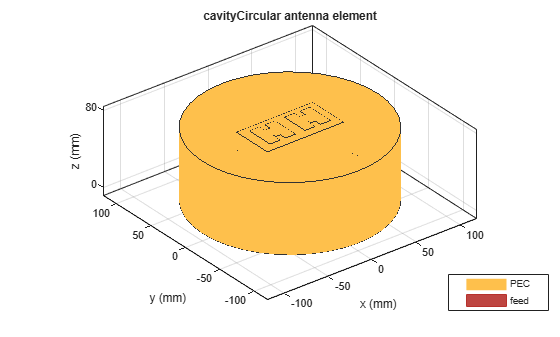Create and visualize a circular cavity-backed cylindrical dielectric resonator antenna.

```e = draCylindrical; ant = cavityCircular(Exciter=e)```
```ant = cavityCircular with properties: Exciter: [1x1 draCylindrical] Substrate: [1x1 dielectric] Radius: 0.1000 Height: 0.0750 Spacing: 0.0750 EnableProbeFeed: 0 Conductor: [1x1 metal] Tilt: 0 TiltAxis: [1 0 0] Load: [1x1 lumpedElement] ```
`show(ant)`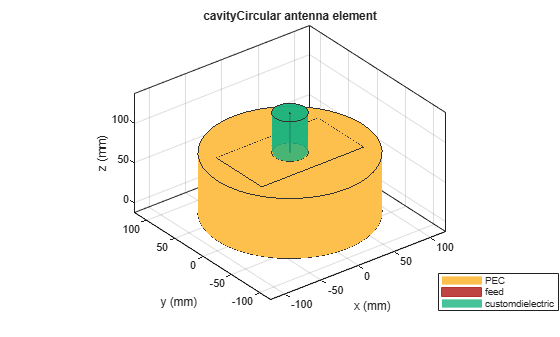This example shows how to create a circular cavity structure as an element in a `conformalArray` and plot its surface current distribution.

Create Circular Cavity Antenna

Create a circular cavity antenna operating at 1 GHz using the `design` function and the `cavityCircular` element from the antenna catalog. Display the antenna.

```f = 1e9; lambda = 3e8/f; ant = design(cavityCircular,f); figure show(ant)```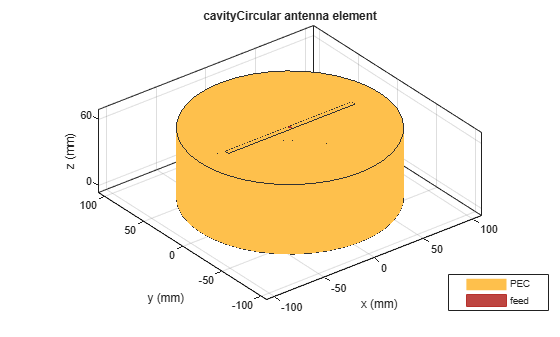Derive Backing Structure

Derive the circular cavity backing structure from the cavity antenna by specifying the `'Exciter'` property as an empty array. Display the backing structure.

```ant.Exciter = []; figure show(ant)```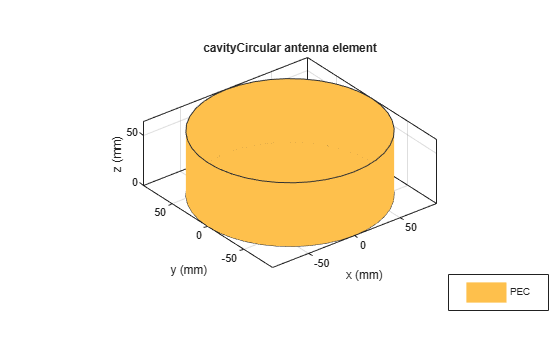Create Conformal Array

Create and display a conformal array with circular cavity as one of its elements.

```ca = conformalArray; ca.Reference = "origin"; ca.ElementPosition = [0 0 0; 0 0 0.25; 0 0 0.5]; ca.Element = {ca.Element{1} ant ca.Element{2}}; figure show(ca)```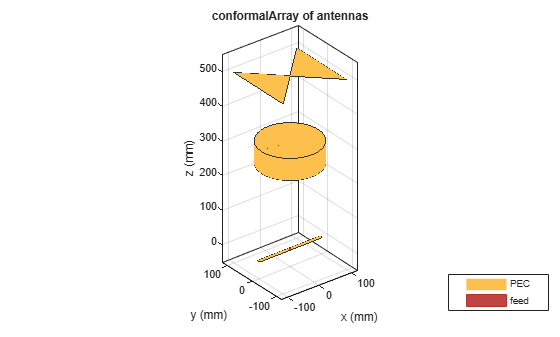Plot Surface Current Distribution

Calculate the current at the feed location and plot the surface current distribution of the conformal array at 1 GHz.

`If = feedCurrent(ca,f)`
```If = 1×2 complex 0.0024 - 0.0005i 0.0029 + 0.0007i ```
```figure current(ca,f,Scale="log10")```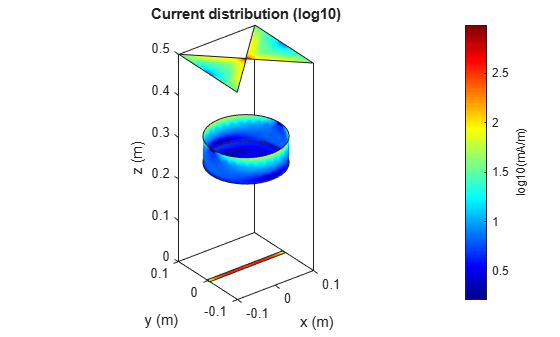## Version History

Introduced in R2017b